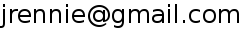# Jason Rennie's Matlab Code

## A Brief Introduction: How to run Fast MMMF

Examples of how to use this code for Fast MMMF: weak.m, strong.m. Unfortunately the data file (marlin.mat) is no longer available.

For the MMMF obj/grad functions, conjgrad returns a vector of all of the parameters, U, V and theta. Here's how to extract:

U = reshape(v(1:n*p),n,p);
V = reshape(v(n*p+1:n*p+m*p),m,p);
theta = reshape(v(n*p+m*p+1:n*p+m*p+n*(l-1)),n,l-1);
Here's how to predict:
X = U*V';
Y = m3fSoftmax(X,theta);
Note that p is the number of columns of U and V and hence the maximum rank of X. I.e. if p is smaller than the smaller dimension of X, then it constrains the rank of X. Ideally, p is set to min(n,m), but, in practice, smaller values are used for efficiency. A value of p=100 was used for my thesis experiments.

## The Code

• Common Code (which you'll need to run many of the below routines)
• Optimization Routines Example usage:
`[w] = conjgrad(randn(d,1),@cgLineSearch,{},@m3fshc,{train,lambda,l},'verbose',1,'tol',1e-6);`
• Line Searches
• Maximum-Margin Matrix Factorization (MMMF)
• MMMF with fixed V (learn U, theta)
• Multiclass Classification Note: this multiclass code sums loss over all classes (not just the max-loss class, as is more common)
Note: these codes are designed for a collaborative prediction task (hence the two-dimensional rating/label matrix). For a more traditional multi-class task, assume n=1 so that Y is a row vector containing the (positive, integer) labels.
• Binary Classification
• Term Frequency Models
• Standard Statistics Distributions
• Regression
• Least squares: ls.m
• Evaluation
• Mean Absolute Error: mae.m
• Zero-one Error: zoe.m
• Maximum Margin Matrix Factorization

## AFS

If you have AFS, you can simply run the following line from within matlab (or add it to your startup.m file):
```addpath '/afs/csail.mit.edu/u/j/jrennie/public_html/matlab'
```

## Other Code

• Naive Bayes
• Classifier Evaluation
• Breakeven - finds threshold that maximizes some function of the classification errors (use with functions below)
• Accuracy - number of correctly labeled examples
• F1-measure - harmonic mean of precision and recall
• R1-measure - harmonic mean of accuracy rate on positive examples (recall) and accuracy rate on negative examples
• Solving Ax=b and Mx=y for x - the Ax=b code assumes that A is symmetric and positive definite; the Mx=y code is simply an interface to the Ax=b code.
• Sparse Diagonal Matrices.
• Precision-based rounding. Select the number of digits of significance to preserve.
• Safe, fast calculation of log-determinant.